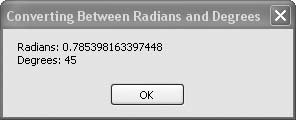# Recipe 6.10. Converting Between Radians and Degrees

#### Problem

You want a simple, consistent, easy-to-read, and easy-to-use way to convert angles between radians and degrees.

#### Solution

Define two constants, RadPerDeg and DegPerRad, and multiply by degrees or radians, respectively, to convert to the other units.

#### Discussion

You can create standalone functions to perform these conversions, but these constants are straightforward definitions, and your code will compile to inline conversions that are compact and fast. The following code defines the constants and uses them to convert a few sample angular values. It's generally best to define your constants at the top of your source-code files or in a global module, but here they are shown close to the code where they are used for easy reference:

` Const RadPerDeg As Double = Math.PI / 180# Const DegPerRad As Double = 180# / Math.PI Dim radians As Double Dim degrees As Double radians = Math.PI / 4# degrees = radians * DegPerRad radians = degrees * RadPerDeg MsgBox("Radians: " & radians.ToString & _ vbNewLine & "Degrees: " & degrees.ToString) `

This code rather redundantly converts radians to degrees and then immediately converts degrees right back to radians. You wouldn't want to do this normally, but it shows both conversions side by side for easy comparison.

Figure 6-10 shows the same angle (45 degrees, or π/4 radians) expressed in the calculated units after conversion using the constants.Both constants are defined using a division calculation. The Visual Basic 2005 compiler converts this math statement to a single constant by doing the division at compile time rather than at runtime, so there is no inefficiency in expressing the constants this way. The value of π is defined as a constant in the Math object with full double-precision accuracy, so the constants defined here are also accurate with Double values.

See "Derived Math Functions" in Visual Studio Help for additional derived functions, many of which assume radian units.Visual Basic 2005 Cookbook: Solutions for VB 2005 Programmers (Cookbooks (OReilly))
ISBN: 0596101775
EAN: 2147483647
Year: 2006
Pages: 400